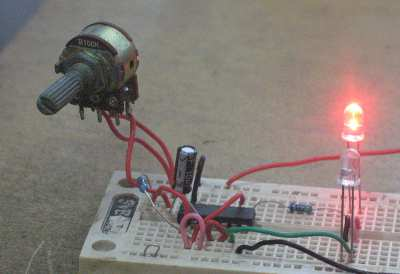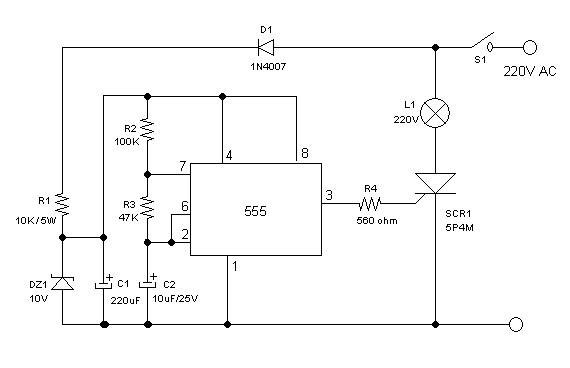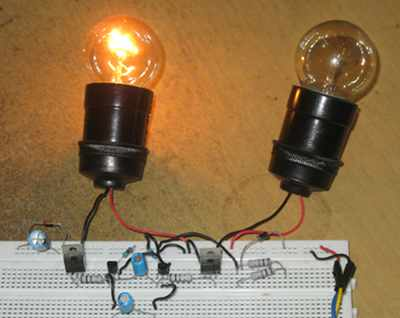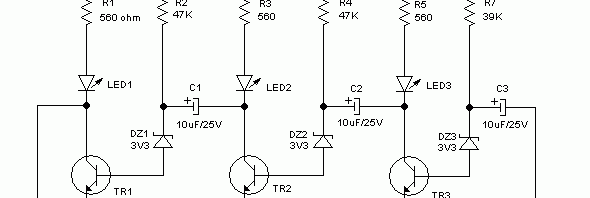## Adjustable Frequency Flip-Flop Circuit Using Inverter GateThis flip-flop circuit uses one potentiometer and one capacitor as the frequency determining components. The assembled circuit is shown in the Figure 1, and the schematic diagram of the circuit is shown in the Figure 2. The circuit consists of one integrated circuit of inverter gates (4069 CMOS or  74C04), one electrolytic capacitor, two resistors, two LEDs, and one potentiometer. […]

## High Voltage 220V AC Lamp Flasher Using 555 ICSimilar with previous high voltage  flip-flop circuit, this circuit controls high voltage (powerline) bulbs (220V AC). Instead of using transistors, this circuit uses NE555 integrated circuit, a very popular chip for timer or oscillator application. The required low voltage supply for 555 IC is provided by direct converter, made up by D1, R1, DZ1, and C1. This low voltage is […]

## High Voltage Flip-Flop With SCR and Low Voltage ComponentsWith only few components, we can use resistors, diodes, and capacitor, we can convert line voltage (220VAC)  directly to low DC voltage (10 VDC). This low voltage powers the low voltage flip-flop standard circuit, but this circuit is then used to drive high voltage SCR. This simple high voltage flip-flop circuit can be used to control alarm lamps, bell, or […]